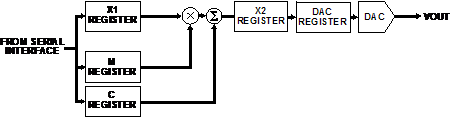# Open-Loop Calibration Techniques for Digital-to-Analog Converters

In principle, you give a digital input to a DAC and it provides an accurate output voltage. In reality, the accuracy of the output voltage is subject to gain and offset errors from the DAC and other components in the signal chain. The system designer must compensate for these errors in order to get an accurate output voltage. This can be implemented with external components and post-manufacture trimming. Digital calibration modifies the input sent to the DAC such that the gain and offset errors are taken into account thus removing the need for external components and trimming.

### Calibration for open-loop systems

Open-loop systems have no feedback path, leaving designers to trust that the output voltage is at the correct value. High accuracy usually requires precision DACs with 14- to 16-bit resolution. The data sheet specifications for integral nonlinearity (INL) and differential nonlinearity (DNL) do not include gain and offset errors. These errors vary from device to device, and must be measured before they can be removed. This is often done as part of a factory calibration.

Figure 1 shows the transfer functions of ideal vs. actual DACs. In this example, the offset error causes a negative output voltage when a 0-V output is expected, and the gain error creates a span greater than that desired.Figure 1. DAC transfer functions

The transfer function can be modeled as a straight line described by y = mx +c.

Where:

y is the output

m is the slope of the transfer function

x is the input to the DAC

c is the offset voltage

An ideal DAC has gain (m) of 1 and offset (c) of 0—and the output tracks the input in a perfectly linear manner. Real DACs have non-ideal gain and offset values that can be compensated once they are known.

Take, for example, a 16-bit DAC with a 0-V to 10-V nominal output span. When the digital input is set to 0, a –30-mV output is measured. When the digital input is set to 65,535 (full scale), a 10.02-V output is measured.

From this, we can determine that:

the offset error is –30 mV

the span error is 20 mV – (–30 mV) = 50 mV, giving an actual span of 10.05V

the gain is 10.05 V/10 V = 1.005

#### Compensating for the Gain Error

To compensate for the gain error, the digital input to the DAC must be multiplied by the reciprocal of the gain error:

x1 = x × (10 V/10.05 V)

where x is the desired input and x1 is the value loaded to the DAC. Inputs of 0 and 65,535 would produce a 10.05-V span, while inputs of 0 and 65,209 will give the desired 10-V span.

#### Compensating for Offset Errors

Offset errors cause the DAC to produce an output voltage other than 0 V for a digital input of 0. The offset error can be measured and removed by adding or subtracting an equivalent digital number to the DAC input.

In this example, the DAC has 65,536 possible codes and an output span of 10.05V. This means that each 1 LSB (least significant bit) step is

10.05 V/65536 = 153.35 µV.

In this example, the –30-mV offset is equivalent to (30 mV/153.35 µV) = 196 LSB. Therefore, a value of 196 written to the DAC will give an output voltage of 0 V.

#### Putting it all together

Compensating the gain and offset errors produces an accurate voltage. The equation below shows how to calculate the correct DAC input to produce the desired voltage.

Actual DAC input = (10 V/10.05 V) × Ideal DAC input + 196

### Alternative Options

Performing these calculations in a DSP or microprocessor requires additional overhead that can be costly and time consuming. Some DACs include on-chip registers that allow the calculations to take place in the DAC, freeing the processor to carry out other functions. The AD536x, AD537x, AD538x, and AD539x families of denseDACmultichannel DACs have 8 to 40 channels with 12- to 16-bit resolution. Single-supply versions can produce 5-V outputs. Dual-supply versions can produce ±10-V outputs. Each device has dedicated m and c registers for each channel, allowing per-channel gain and offset calibration.

Figure 2 shows one channel of the AD5370 16-bit, 40-channel DAC. Calculating the values for m and c registers is described in the following steps:

1. Measure the offset and full-scale errors by setting the DAC input to zero and full scale.
2. Calculate the actual LSB size by dividing the span by the number of possible codes (65536 in this case)
3. Subtract the number of LSBs corresponding to the excess span from the default m register value. For example, an excess span of 50 mV in a 10 V range corresponds to 326 LSBs
4. Add the number of LSBs corresponding to the offset to the default c register value. For example, an offset of –10 mV is 65 LSBs

The DAC can now be treated as if it were ideal and will calculate appropriate value to compensate for internal and system errors.Figure 2. Single channel of AD5370 DAC